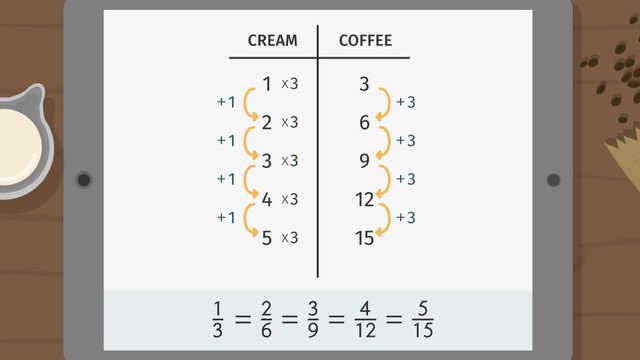# Ratio Tables - Additive and Multiplicative StructureRating

Ø 5.0 / 2 ratings
The authorsTeam Digital

## Basics on the topicRatio Tables - Additive and Multiplicative Structure

After this lesson, you will be able to use the additive and multiplicative structure of ratio tables to find missing entries in a ratio table.

The lesson begins by teaching you what a ratio table is. It leads you to learn how to identify its additive and multiplicative structures. It concludes with showing how to find missing entries in a ratio table.

Learn about the additive and multiplicative structures of ratio tables by observing how Basti creates the concoction berry brew blend!

This video includes key concepts, notation, and vocabulary such as ratio table (a structured set of equivalent ratios); row (the horizontal entries in a table); column (the vertical entries in a table); reduced ratio (a ratio in the simplest form such that the GCF of the numerator and the denominator is 1); additive structure (the pattern in a ratio table when entries are inspected by column); multiplicative structure (the pattern in a ratio table when entries are inspected by row); and reciprocal (the multiplicative inverse of a number).

Before watching this video, you should already be familiar with ratios and equivalent ratios.

After watching this video, you will be prepared to learn how to solve real-world and mathematical problems involving ratios and rates such as unit rate problems, percentage problems, and conversion of measurement units.

Common Core Standard(s) in focus: 6.RP.A.3.A A video intended for math students in the 6th grade Recommended for students who are 11 - 12 years old